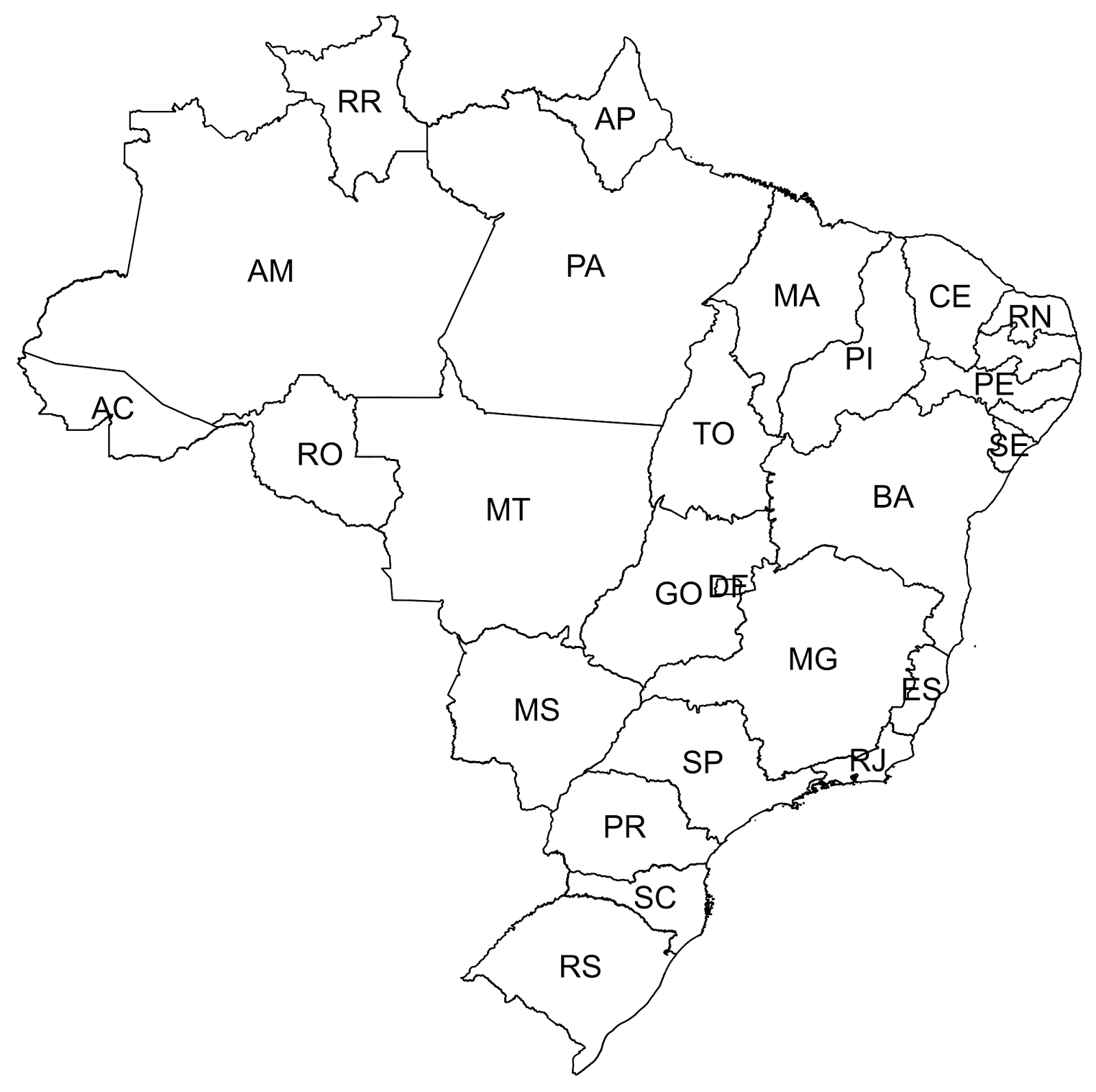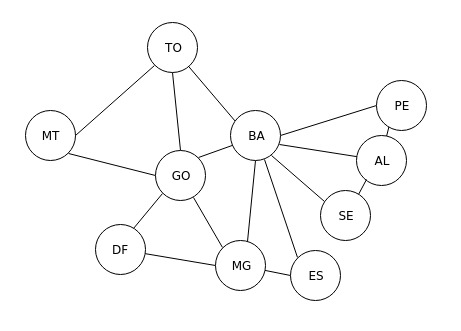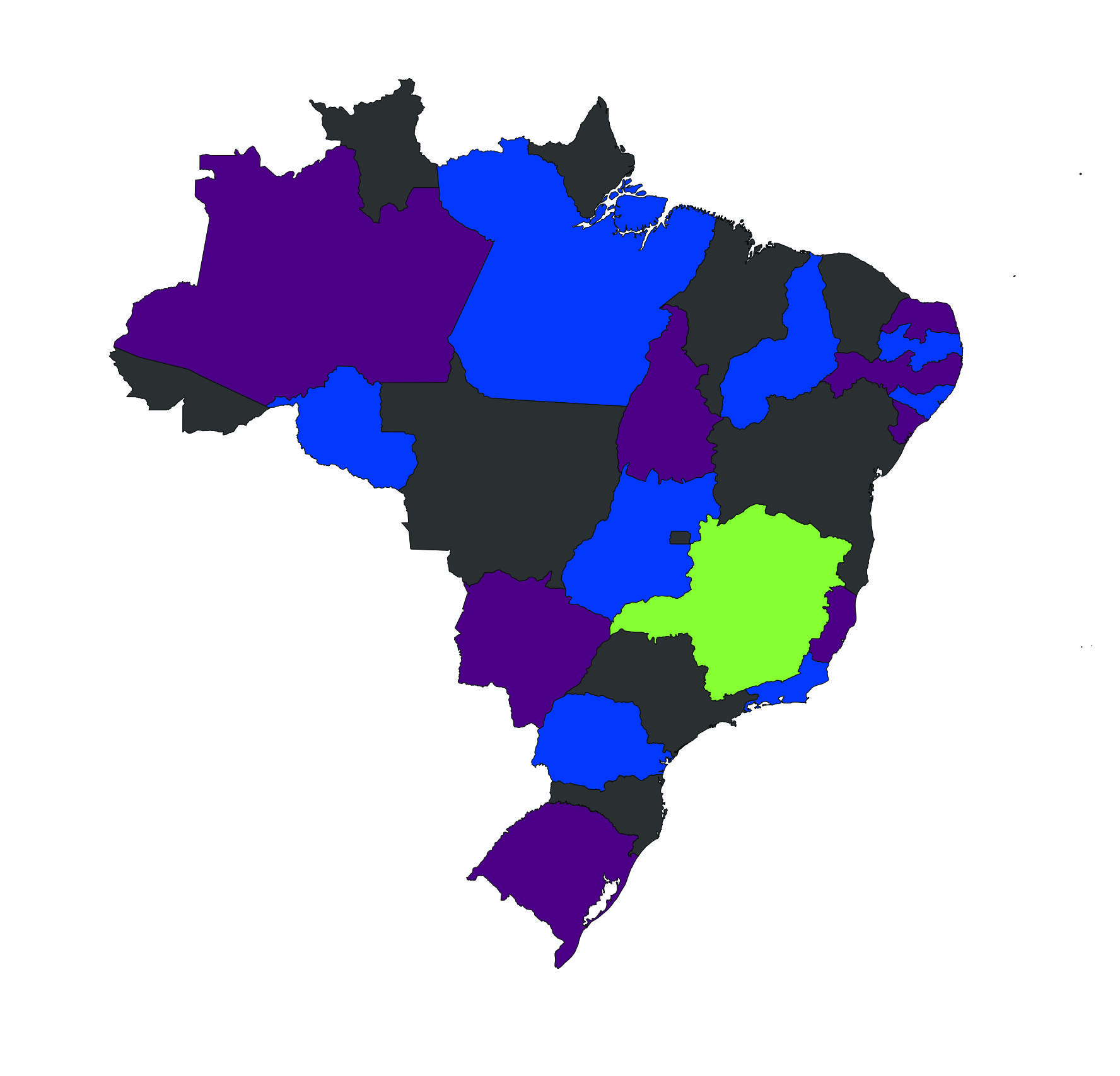# Four color theorem with Prolog

On this post I’ll talk about the four color theorem and how to solve it using Prolog, this is a very common exercise when you start studying Prolog and you can find a lot of examples on the internet with small maps, this examples can be easily replicated to large maps, on this example I’ll use the map of Brazil that contains 27 federative units.

## Four Color Theorem

How many different colors you think are sufficient to color a map considering that you can’t use the same color on two adjacent regions? Let’s imagine that you want to color the world map with this rule, how many pencils do you need? This is already solved and you need only four colors! This is the four color theorem.

“In mathematics, the four color theorem, or the four color map theorem, states that, given any separation of a plane into contiguous regions, producing a figure called a map, no more than four colors are required to color the regions of the map so that no two adjacent regions have the same color. Adjacent means that two regions share a common boundary curve segment, not merely a corner where three or more regions meet.”

A good curiosity is that four color theorem can only be proved by computers.

Example:### Solving

To solve it, as the title of the text describes, we will use Prolog a programming language that applies the logic paradigm of programming, I already wrote about an if you are interested you can check here.

#### Brazil Map

We will apply this theorem on Brazil map, I chose the Brazil map because, with 27 federative units, it can be considered a large map, but the algorithm that we’ll be used can be replicated to any map.#### Solving the Problem

The first thing that we can do is to transform Brazil map in a graph to see more clearly which states are adjacent.After this we already know our facts that Prolog need to solve our problem, the first thing that we can do is to choose four colors to paint our map, just declare the facts:

```color(black).
color(byzantine).
color(sapphire_blue).
color(screamin_green).
```

After this the next thing that we can do is to write the rule of two adjacent states, this rule needs to tell Prolog that two states can’t have the same color.

```adjacent(State1Color, State2Color) :-
color(State1Color), color(State2Color),
State1Color \= State2Color.
```

Explaining the code: On the first part of the rule we declare that the rule names are `adjacent` and that it will receive two states as params called `State1Color` and `State2Color`, after this, we give a color for states and a rule that these colors can’t be the same.

This rule is simple, it receives two states, give a color to them respecting the rule that colors can’t be the same. With all these steps done we can write all the adjacent states as facts.

We can test our rule on some region of our graph, for this, I chose these regions that in my opinion is a complex region:Now, just make a query asking for Prolog solve it using or adjacent rule:

```?- adjacent(TO, BA), adjacent(TO, GO), adjacent(TO, MT),
```

And the output result will be:

```AL = ES, ES = GO, GO = sapphire_blue,
BA = DF, DF = MT, MT = byzantine,
MG = PE, PE = SE, SE = TO, TO = black
```

After this, we can paint our graph and conclude that it is correct:Ok, now we know that our rule is working as expected, we have two options or we make a query passing all adjacent states (like the one that we did before but all states), or we code a rule and just call this rule as a query. I think that the first option is equal to the previous example, so let’s create a rule to paint the Brazil map, this rule is very simple, it’s only map all adjacent states and put inside a rule:

```brazil(AC, AM, PA, RR, RO, AP, MT,
MA, TO, GO, MS, DF,
PI, CE, RN, PB, PE, AL,
SE, BA, ES, MG, RJ,
SP, PR, SC, RS) :-
```

This rule only receive 27 federation units, map them as adjacent and will output the colors, now we just need to make a query passing the states on the correct order:

```brazil(AC, AM, PA, RR, RO, AP, MT,
MA, TO, GO, MS, DF,
PI, CE, RN, PB, PE, AL,
SE, BA, ES, MG, RJ,
SP, PR, SC, RS).
```

And the first result output will be:

```AC = AP, AP = BA, BA = CE, CE = DF, DF = MA, MA = MT, MT = RR, RR = SC, SC = SP, SP = black,
AL = GO, GO = PA, PA = PB, PB = PI, PI = PR, PR = RJ, RJ = RO, RO = sapphire_blue,
AM = ES, ES = MS, MS = PE, PE = RN, RN = RS, RS = SE, SE = TO, TO = byzantine,
MG = screamin_green
```

After some minutes painting our map, I got:The map is correct and a fun fact about this solution is that only one state needs `screamin_green` color.

Our experiment is done, but another question can be answered now, how many different solutions it has? We only used the first solution that Prolog has given to us. Fortunately, we have a way to calculate it, just use the predicate `aggregate_all/3` and it will count how many results it finds.

```?- aggregate_all(count, brazil(AC, AM, PA, RR, RO, AP, MT, MA, TO, GO, MS, DF, PI, CE,
RN, PB, PE, AL, SE, BA, ES, MG, RJ, SP, PR, SC, RS), Count).
```

And the result is huge:

```Count = 384860160.
```

three hundred eighty-four million, eight hundred sixty thousand, one hundred sixty of combinations found.

And finally, we finished our experiment.

### Conclusion

As I said in my last post about logic programming here, Prolog is worth learning, and for solving this kind of problem it shines but you’ll need to expend a lot of time giving the facts (as we did with adjacent states).